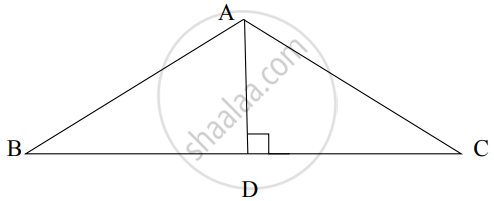# From the given figure, in ∆ABC, if AD ⊥ BC, ∠C = 45°, AC = 82 , BD = 5, then for finding value of AD and BC, complete the following activity.Activity: In ∆ADC, if ∠ADC = 90°, ∠C = 45° ......[Given] - Geometry

Sum

From the given figure, in ∆ABC, if AD ⊥ BC, ∠C = 45°, AC = 8sqrt(2) , BD = 5, then for finding value of AD and BC, complete the following activity.∴ ∠DAC = square   .....[Remaining angle of ∆ADC]

By theorem of 45° – 45° – 90° triangle,

∴ square = 1/sqrt(2) AC and square = 1/sqrt(2) AC

∴ AD =1/sqrt(2) xx square and DC = 1/sqrt(2) xx 8sqrt(2)

∴ AD = 8 and DC = 8

∴ BC = BD +DC

= 5 + 8

= 13

#### Solution

∴ ∠DAC = 45°    .....[Remaining angle of ∆ADC]

By theorem of 45° – 45° – 90° triangle,

∴ AD = 1/sqrt(2) AC and DC = 1/sqrt(2) AC

∴ AD =1/sqrt(2) xx 8sqrt(2) and DC = 1/sqrt(2) xx 8sqrt(2)

∴ AD = 8 and DC = 8

∴ BC = BD +DC

= 5 + 8

= 13

Concept: Property of 30°- 60°- 90° Triangle Theorem
Is there an error in this question or solution?

Share